# Squeeze the Memory Consumption of Deep Learning¶

One important theme about deep learning is to train deeper and larger nets. While the hardware has been upgraded rapidly in recent years, the huge deepnet monsters are always hungry about the GPU RAMS. Being able to use less memory for the same net also means we can use larger batch size, and usually higher GPU utilization rate.

This article discusses how memory allocation optimization can be done for deep neural nets, and provide some of candidate solutions to the problems. The solutions discussed in this article is by no means complete, but rather as example that we think is useful to most cases.

## Computation Graph¶

We will start the discussion by introducing computation graph, since this is the tool that will help us in the later part of the section. Computation graph describes the (data-flow) dependencies between the operations in the deep nets. The operation performed in the graph can either be fine-grained or coarse grained. The following figure gives two examples of computation graph.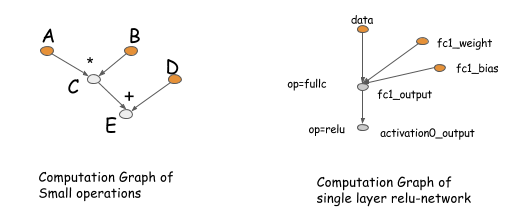The idea of computation graph is deeply rooted in the packages such as Theano, CGT. Actually they also exists implicitly in most libraries as the network configuration. The major difference in these library comes to how do they calculate gradient. There are mainly two ways, doing back-propagation on the same graph, or have an explicit backward path that calculates the gradient needed.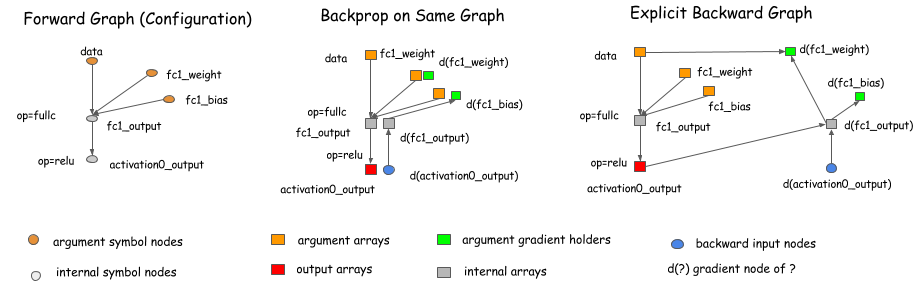Libraries like caffe, cxxnet, torch uses the backprop on same graph. While libraries like Theano, CGT takes the explicit backward path approach. We will adopt the explicit backward path way in the article, because it brings several advantages in turns of optimization.

However, we should emphasize that choosing the explicit backward path way for execution will not restrict us to scope of symbolic libraries such as Theano, CGT. We can also use the explicit backward path for gradient calculation of layer-based(which ties forward, backward together) libraries. The following graph shows how this can be done. Basically, we can introduce a backward node that links to the forward node of the graph, and calls the layer.backward in the backward operations.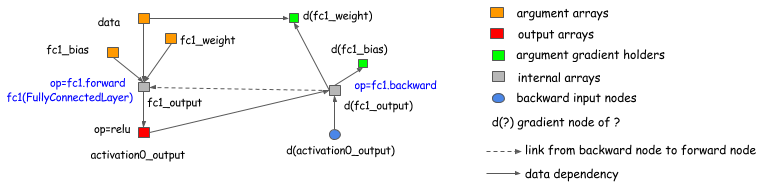So this discussion applies to almost all deep learning libraries that exists (There are differences between these libraries, e.g. high order differentiation. which are beyond the scope of in this article).

Why explicit backward path is better? Let us explain it with two examples. The first reason is that the explicit backward path clearly describes the dependency between the computation. Consider the following case, where we want to get the gradient of A and B. As we can see clearly from the graph, that computation of d(C) gradient do not depend on F. This means we can free the memory of F right after the the forward computation is done, similarly the memory of C can be recycled.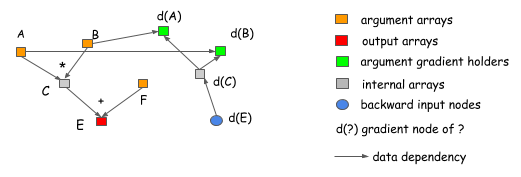Another advantage of explicit backward path is to be able to have a different backward path rather than an mirror of forward one. One common example is the split connection case, as shown in the following figure.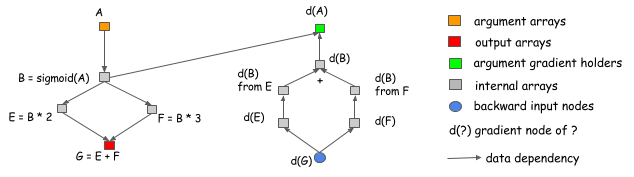In this example, the output of B is referenced by two operations. If we want to do the gradient calculation in the same network, an explicit split layer need to be introduced. This means we need to do the split for the forward pass as well. In this figure, the forward pass do not contain a split layer, but the graph will automatically insert a gradient aggregation node before passing gradient back to B. This helps us to save the memory cost of allocating output of split layer, as well as operation cost of replicate the data in forward pass.

If we adopt the explicit backward view of computation graph, there is no difference between the forward pass and backward pass. We will simply go forward in topological order of the computation graph, and carry out computations. This also simplifies our discussions. The problem now becomes:

• How to allocate the memory of each output node of a computation graph?

Hmm, seems it has nothing to do with deep learning, but more of context of compiling, data flow optimization etc. But it is really the hungry monster of deep learning that motivates us attack this problem, and benefit from it.

## What can be Optimized¶

Hopefully you are convinced that the computation graph is a good way to discuss memory allocation optimization techniques. As you can see some memory saving can already been bought by using explicit backward graph. Let us discuss more about what optimization we can do, and what is the baseline.

Asumme we want to build a neural net with n layers. A typical implementation of neural net will need to allocate node space for output of each layer, as well as gradient values for back-propagation. This means we need roughly 2 n memory cells. This is the same in the explicit backward graph case, as the number of nodes in backward pass in roughly the same as forward pass.

### Inplace Operations¶

One of the very first thing that we can do is inplace memory sharing of operations. This is usually done for simple operations such as activation functions. Consider the following case, where we want to compute the value of three chained sigmoid function.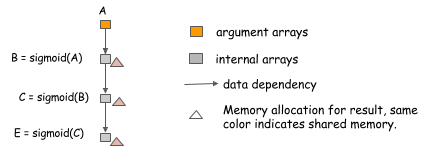Because we can compute sigmoid in the inplace manner, that is, use the same memory for input and output. We can simply allocate one copy of memory, and use it compute arbitrary length of sigmoid chain.

However, the inplace optimization sometimes can be done in the wrong way, especially when the package tries to be a bit general. Consider the following case, where the value of B is not only used by C, but also F.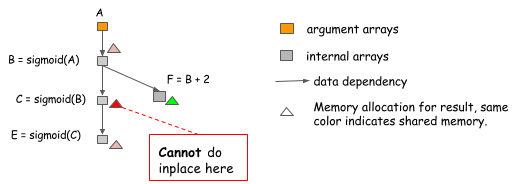We cannot perform inplace optimization because the value of B is still needed after C=sigmoid(B) is computed. So an algorithm that simply do inplace optimization for every sigmoid operation might fall into such trap, and we need to be careful on when we can do it.

### Normal Memory Sharing¶

Memories can also be shared besides the inplace operation. Consider the following case, because the value of B is no longer needed when we compute E, we can reuse the memory to hold the result of E.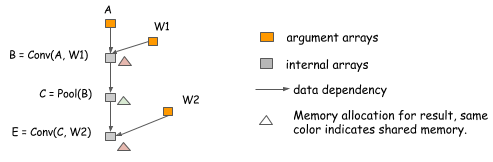We would like to point out that is ***memory sharing does not necessarily require same data shape****. In the above example, the shape of B and E can be different, and we can simply allocate a memory region that is the maximum of the two sizes and share it between the two.

### Real Neural Net Allocation Example¶

The above examples are all make up cases, that only contains the computation of the forward pass. Actually the idea holds the same for the real neural net cases. The following figure shows an allocation plan we can do for a two layer perception.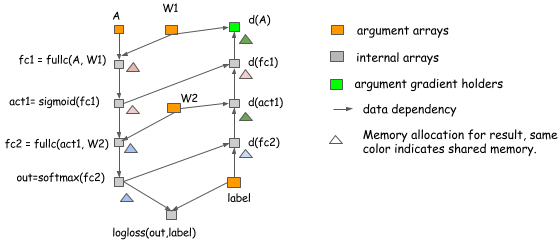In the above example:

• Inplace optimization is applied on computing act1, d(fc1), out and d(fc2).
• The memory sharing is used between d(act1) and d(A).

## Memory Allocation Algorithm¶

We have discussed how the general techniques to optimize memory allocations in previous section. However, we also see that there are traps which we want to avoid like the inplace case. How can we allocate the memory correctly? This is not a new problem. For example, it is very similar to register allocation in compilers. So there could be a lot we can borrow. We do not attempt to give a comprehensive review of techniques here, but rather introduce some simple but useful trick to attack the problem.

The key problem is we want to place resources, such that they do not conflict each other. More specifically, each variable have a life time between the time it get computed till the last time it get used. In the multilayer perception case, the life time of fc1 ends after act1 get computed.The principle is to only allow memory sharing between the variables whose lifetime do not overlap. There are multiple ways to solve this problem. One possible way is to construct the conflicting graph of with each variable as node and link edge between variables with overlapping lifespan, and run a graph-coloring algorithm. This will likely require $$O(n^2)$$ complexity where n is number of nodes in the graph, which could be an reasonable price to pay.

We will introduce another simple heuristic here. The idea is to simulate the procedure of traversing the graph, and keep a counter of future operations that depends on the node.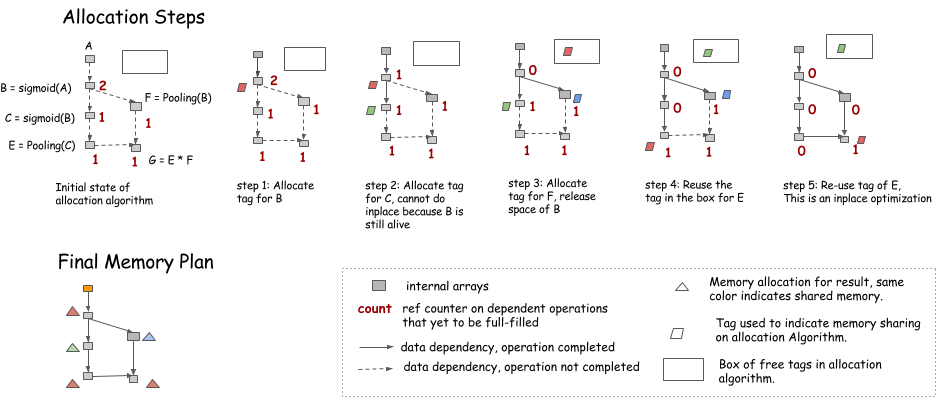• An inplace optimization can be performed when only current operation depend on the source(i.e. counter=1)
• A memory can be recycled into the box on the upper right corner when counter goes to 0
• Every time, when we need new memory, we can either get it from the box, or allocate a new one.

One note is that during the simulation, no memory is allocated, but we rather keep record of how much memory each node need, and allocate the maximum of the shared parts in the final memory plan.

### Static vs Dynamic Allocation¶

If you think carefully, you will find the above strategy exactly simulates the dynamic memory allocation procedure in imperative languages such as python. The counter is the reference counter of each memory object, and the object get garbage collected when the reference counter goes to zero. In that sense, we are simulating the dynamic memory allocation once to create a static allocation plan. Now the question is, can we simply use an imperative language that dynamically allocates and de-allocates memories?

The major difference is that the static allocation is only done once, so we can afford to use more complicated algorithms

• For example, do searching over memories sizes that are similar to the require memory block.
• The allocation can also be made graph aware, see more discussion in next section.
• The dynamic way will push more pressure on fast memory allocator and garbage collector.

There is also one takeaway for users who want to reply on dynamic memory allocations: do not take unnecessary reference of object. For example, if we organize all the nodes in a list and store then in a Net object, these nodes will never get de-referenced, getting us no gain of the space. Unfortunately, this is one common way to organize the code.

## Allocation for on Parallel Operations¶

In the previous section, we discussed how we can simulate the running procedure of computation graph, and get a static allocation plan. However, there are more problems when we want to optimize for parallel computation as resource sharing and parallelization are on the two ends of a balance. Let us look at the following two allocation plan for the same graph: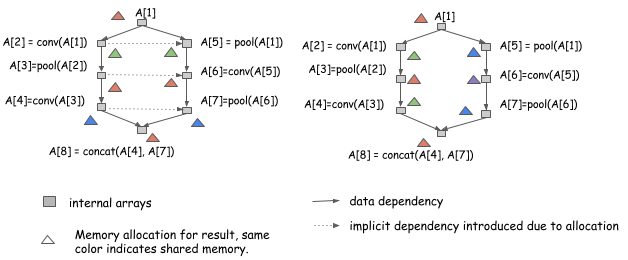Both allocation plans are valid, if we run the computation in a serial manner from A to A. However, the allocation plan on the left side introduces extra dependencies, which means we cannot run computation of A and A in parallel, while the right one can.

As we can see that if we want to parallelizing the computation, more care need to be done in terms of computation.

### Stay Safe and Correct First¶

Stay correct, this is the very first principle we need to know. This means execute in a way to take the implicit dependency memory sharing into consideration. This can done by adding the implicit dependency edge to execution graph. Or even simpler, if the execution engine is mutate aware as described in the dependency engine note, push the operation in sequence and write to the same variable tag that represents the same memory region.

Another way is always produce memory allocation plan that is safe, which means never allocate same memory to nodes that can be parallelized. This may not be the ideal case, because sometimes memory reduction is more desirable, and there is not too much gain we can get by multiple computing stream execution on the same GPU.

### Try to Allow More Parallelization¶

Given that we can always be correct, we are now safe to do some optimizations. The general idea is to try to encourage memory sharing between nodes that cannot be parallelized. This again can be done by creating a ancestor relation graph and query this during allocation, which cost around $$O(n^2)$$ time to construct. We can also use heuristic here, for example, one way is to color the path in the graph. The idea is shown in the figure below, every time we tries to find a longest path in the graph, color them to same color, and continue.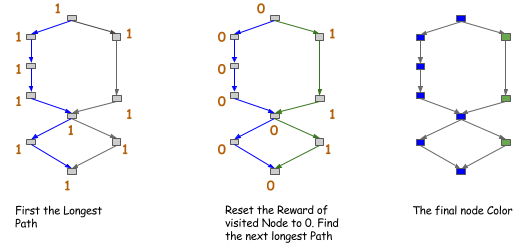After we get the color of the node, we can only allow sharing (or encourage such sharing ) between nodes in the same color. This is a more strict version than the ancestor relation, but only cost $$O(n)$$ time if we only search for first k path.

The strategy discussed here is by no means the only solution, we can expect more sophisticated approaches along this line.

## How much can We Save¶

Thanks for reading till this part! We have discussed the techniques and algorithms we can use to squeeze the memory usage of deep learning. Now comes the question on how much we can really save by using these techniques.

The answer is we can roughly reduce the memory consumption by half using these techniques. This is on the coarse grained operation graphs that are already optimized with big operations. More memory reduction could be seen if we are optimizing a fine-grained computation network used by symbolic libraries such as Theano.

Most of the ideas in this article inspires the design of mxnet. We provide an Memory Cost Estimation Script, which you can play with to see how much memory we need under different strategies.

If you play with the script, there is one option called forward_only, which shows the cost only running the forward pass. You will find that the cost is extremely low compared to others. You won’t be surprised if you read previous part of the article, this is simply because more memory re-use if we only run the forward pass. So here are the two takeaways:

• Use computation graph to allocate the memory smartly and correctly.
• Running deep learning prediction cost much less memory than deep learning training.

## Contribution to this Note¶

This note is part of our effort to open-source system design notes for deep learning libraries. You are more welcomed to contribute to this Note, by submitting a pull request.